# Buffon's Needle Problem

### Scott E. Brodie 5/22/1999

Children all over the world (and no doubt many grownups, too) play at "lines and squares", attempting to avoid stepping on the joints or cracks between the panels of pavement in the sidewalk. In this note, we will explore a randomized, mathematical version of the game. The idea was first raised by Georges Louis Leclerc, Comte de Buffon in his paper, Sur le jeu de franc-carreau, published in 1777.

Buffon first considered the case of a small coin ("un ecu") thrown at random on a floor tiled with congruent squares. It was apparently then all the rage to bet as to whether the coin would land entirely within the boundaries of a single tile ("à franc-carreau"), or would land across one of the boundaries between two adjacent tiles. Buffon was apparently the first to realize that the fair odds for such a wager could readily be determined by noting that the coin would land entirely within a square tile whenever the center of the coin landed within a smaller square, whose side was equal to the side of a floor tile less the diameter of the coin:Clearly, the probability that the coin lands wholly within a single tile is simply the ratio of the area of the tile to the area of the square contained within the dashed lines, as the center of the coin is equally likely to land in any two regions of equal area, or, more generally, the probability that the center of the coin lands in one of two regions equals the ratio of the areas of the two regions.

This seems to have been the beginning of the study of "geometric probability," where probabilities are determined by comparison of measurements, rather than by identifying and counting alternative, equally probable discreet events, such as specific hands of cards, or rolls of dice.

Buffon then raises the question of a more interesting case -- suppose one throws, not a circular object, but an object of a more complex shape, such as a square, a needle, or a "baguette" (a rod or stick). He treats in detail the famous "Needle Problem": Suppose a needle is thrown at random on a floor marked with equidistant parallel lines. What is the probability that the needle will land on one of the lines?

Ever helpful, Buffon points out that "On peut jouer ce jeu sur un damier avec une aiguille à coudre ou une épingle sans tête." (You can play this game on a checkerboard with a sewing-needle or a pin without a head.)

It is instructive to start with a simpler problem: what is the probability that a disk of diameter d will land on one of the lines, where the lines are separated by a distance h?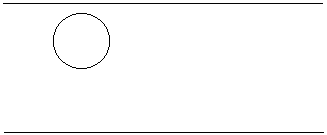As in the case of square tiles, it can be seen by inspection that the disk will land between the lines whenever the center of the disk lands in a band of width h - d. Thus, the probability that a disk of diameter d lands between the lines is ( h - d ) / h, and the probability that the disk lands on a line is simply d /h.

Now consider the case of the needle thrown on the floor ruled with equidistant parallel lines. For simplicity, suppose that the length of the needle is equal to the spacing, h, between adjacent lines.

For a moment, let's restrict our attention to those throws when the needle falls perpendicular to the lines on the floor. In this case, the needle acts just like a disk of diameter h as in the previous example -- the probability that the needle crosses a line is h / h = 1. Similary, if the needle falls parallel to the ruled lines, the probability that it crosses a line is zero.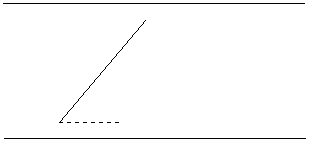Now consider the case of a needle which falls so as to make an angle, θ, with the parallel lines on the floor. Then reference to the figure confirms that in this case, the needle has an effective "height" of h sin θ, and so the probability that the needle in this orientation will cross a line is h sin θ / h = sin θ. Of course, this formula includes the special cases noted above where the needle falls perpendicular or parallel to the lines on the floor.

In order to determine the overall probability that the needle lands on a line, we must account for the probability of each possible orientation of the needle, and then apply our formula for the probability that the needle, in that orientation, lands so as to cross a line on the floor. This sort of calculation is an application of the notion of "conditional probability," which is explained on a separate page.

In the present context, the gamut of possible orientations of the needle runs from θ = 0° to θ = 180°, as nothing changes if the needle is reversed end-for-end. We can partition this range of possible orientations into a collection of non-overlapping small intervals, say {Θi}. Denote by Pi) the probability that a needle lands with orientation in the interval Θi. Since all orientations of the needle are equally likely, the probabilities Pi) are simply given by allocating to each interval Θi a probability proportional to its length. Since the sine function is continuous, if every interval Θi is small enough, we may pick a single representative value,  θi , within the interval, Θi, such that sin θi is an adequate approximation to the conditional probabity that a needle, falling with any orientation in the interval Θi, will cross a line. In order to obtain the overall probability that a needle tossed at random crosses a line, we sum over the possible orientations Θi, and take the limit as the partition {Θi} is indefinitely refined :

P(needle lands on a line) = lim ∑ sinθi Pi).

Limits of this form arise frequently. In fact, this limit is simply the average of the function sin  θ over the interval from θ = 0° to θ = 180°. Details are given on a separate page on how to compute the average of function.

There are several ways to compute the average of sin θ. Here is a geometrical approach:

Consider the unit circle (the circle who's radius is 1), with, say, n radial "spokes" drawn at equal intervals from θ = 0° to θ = 360°. The angle between adjacent spokes is 360°/n.

Now consider a regular polygon of n sides. If the segments connecting the center of the polygon to each vertex are drawn, there result n triangles, so the sum of the angles of the polygon is n·180° - 360° = 180°·(n - 2). Apportioning this sum to the n angles of the regular polygon, we see that each angle must be 180°·(n - 2)/n. Then the exterior angle at each vertex is 180° - 180°·(n - 2)/n = 180°(1 - (n - 2)/n) = 180°·2/n = 360°/n,

which is the same as the angle between adjacent spokes back on the unit circle.

This means that the n spokes of the unit circle can be rearranged to form a regular polygon of n sides, each of length 1, as shown in the figure: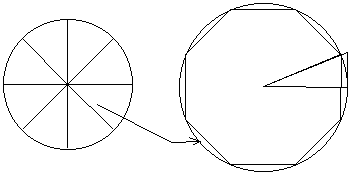Now as n increases, the size of this regular polygon increases as well. Denote the radius of the circle which circumscribes this polygon by rn and the circumference of this circle by cn. Of course, cn = 2πrn.

If we are to estimate the average of sin θ, we must first add up the sines of the angles ranging from 0° to 180° - that is, the vertical components of the vectors represented by the spokes of the top half of the unit circle. As we have just seen, we can first rearrange these vectors end-to-end to form the right-hand half of the corresponding regular polygon. But the sum of the vertical components of these vectors nearly equals the diameter of the circumscribing circle! Thus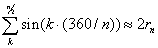dividing by n / 2, and then taking the limit as n →∞ yields the average we are seeking.

It would appear from the figure that, as n becomes large, the regular polygon of n sides and its circumscribing circle become more nearly coincident. In particular, it seems plausible that as n →∞, rn = cn →n. If this approximation is sufficiently accurate, we could then compute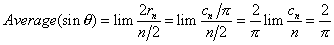In order to more rigorously understand the dependence of cn on n, consider the circular sector with radius rn and central angle u = 180°/n (half of the central angle of the regular polygon of n sides, each of length 1.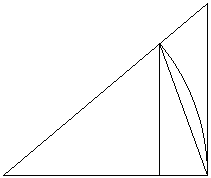The area of this circular sector is (1/2)rn(cn/2n). Next, inscribe an isosceles triangle in this circular sector: if we take one of the sides of length rn as base, the corresponding altitude has length rn·sin u = 1/2, since each side of the regular polygon is of length 1. Thus the area of this triangle is (1/2)rn(1/2) = rn/4. On the other hand, the larger right triangle determined by this sector, with base of length rn and altitude rn·tan u has area

(rn² tan u) / 2 = rn / 4cos u

since rn·sin u = 1/2. Since the isosceles triangle is a subset of the sector, which in turn is a subset of the larger right triangle, the areas are related by the inequalities:

Area (isosceles triangle) ≤ Area(sector) ≤ Area (right triangle)

or

rn / 4  ≤  rncn / 4n  ≤  rn / 4cos u.

Cancelling the common factor of rn /4, we obtain

1 ≤  cn / n  ≤  1 / cos u.

Taking limits as n increases indefinitely, u approaches 0, cos u approaches 1, and we verify that limn→∞ cn / n = 1. We may now calculate with confidence as above.

Referring back to the Needle Problem, we conclude that

Probability (Needle lands on a line) = 2/π.

It was pointed out in the last century that rearranging this equation to the form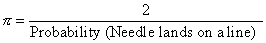provides an amusing way to estimate the number π. Of course, in the modern era, there is no need to spend hours with a needle and a tally sheet. It is a simple exercise to write a computer program to simulate the dropping of the needle and the tabulation of the results. Any of the following links will allow you to simulate the experiment for yourself (see also some remarks):

### Remarks:

1. The calculation of the average of sin θ above was deliberately phrased in terms of angles measured in degrees rather than radians to emphasize that the appearance of the number π in the result is not an artifact of the choice of units for the angular measure, but arises from the circular symmetry of the possible orientations of the needle.

2. Author's Query: We have shown how the probability of a needle landing between the cracks on the floor is determined by the average of the function sin θ, or, what is essentially the same thing, the area under the curve y = sin θ. This curve is usually termed a "sinusoid". In Buffon's original paper, he describes his calculation as relating the probability to "l'aire d'une partie de la cycloïde ...". Clearly, the curve which we now refer to as a "cycloid" is not what is called for. Can any reader provide information as to whether Buffon uses the term "cycloïde" to mean what we now call a "sinusoid", or otherwise clarify this passage?

Note:Twelve and a half years after this article has been published, at the very beginning (January 4) of 2013, Scott Brodie has received a note from Professor Jordan Ellenberg of University of Wisconsin with an attachement that included an article Studies in the history of probability and statistics XXXIX Buffon's cycloid by Philip Holgate (Biometrika, Vol. 68, No. 3 (Dec., 1981), pp. 712-716). It appears from the article that Scott's question has been raised several times in the past. The author of the article speculates as to why Buffon might have set the problem up in terms of the cycloid rather than in terms of the integral of sin(x).

3. A few alternative approaches to the calculation of Average(sin θ) seem worth mention:

If we permit ourselves the use of complex exponentials, the geometric argument given above can be rephrased algebraically: The vector sum of the spokes of the top half of the unit circle can be written as a finite geometric series,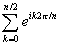.

But the first term of the series is clearly equal to 1, the last term is equal to -1 (at least so long as we keep n even), and the ratio of successive terms is ei/n. Thus, the sum of the series is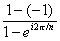.

To obtain the average, divide by n/2 and take the limit as n →∞ (recalling that for small x, we may use the approximation ex → 1 + x):.

The vertical component of the vector average is equal to the imaginary part of this result.

Another cute trick was suggested by Heinrich Dorrie in his treatment of the Buffon Needle Problem in his classic text 100 Great Problems of Elementary Mathematics : Their History and Solution. Recall first a classic "Theorem of Pappus": the area of a "surface of revolution" swept out by an arc revolved about a line which does not intersect it is equal to the product of the length of the arc and 2π times the average (with respect to distance along the arc) of the distance from the line to the arc. In the case of the unit sphere (the sphere of radius 1), the surface is swept out by a single meridian (of length π), rotated about the axis of the sphere. Since the distance from the axis to a point on the meridian is just sin θ (where θ is measured from the axis of the sphere), and the area of the sphere is , we have

4π = 2π·π·Average(sin θ), or

Average(sin θ) = 2/π.

Of course to apply this method, one needs an independent calculation of the area of a sphere. One nice approach is suggested by the following figure:The diagram is a cross-section of a sphere and its enclosing cylinder. The narrow strip of the spherical surface between the dotted lines may be approximated by a piece of conical surface, with radius y and slant-height s. The area of this strip of conical surface is 2·π·s·y. But by similar triangles, h/y = s/r  or hr = sy. But hr is the area of the strip of the enclosing cylinder which lies between the dotted lines. Thus, each strip of the sphere has the same area as the corresponding strip of the cylinder, so the total area of the sphere must equal the total (lateral) area of the cylinder, which is just r·2r = 4πr2.

Archimedes himself proposed another argument: having determined the volume of a sphere to be 4/3 · πr3 by a "mechanical" argument, he observed that, since one can envision cutting apart a sphere into an array of pyramidal components, whose height is the radius of the sphere, and whose bases sum to the surface area of the sphere (much like one can cut an orange into wedges, and then flatten the rind of each wedge, breaking the orange segments into pyramidal fragments), the volume must equal the product 1/3 · r · (Area of sphere),  since the volume of a pyramid is one third the product of its height and the area of its base. Thus the area of a sphere must ber2.

Of course, the problem of finding the average value of a function is a basic result in integral calculus. The methods of calculus show directly that the area under an arch of the sine curve (from 0 to π) is 2. Thus the average value of sin θ is simply 2 /π.

4. A derivation of a completely different sort along with a Java simulation can be found on a separate page.

5. #### Remarks on Buffon Needle Problem simulation programs.

For simplicity, assume that the length of the needle and the separation of the lines on the floor are both equal to 1.

A program to simulate the Buffon Needle Problem usually begins with a random number generator, which supplies two random numbers for each "throw" of the needle: one to indicate, say, the distance from a line on the floor to the "lower" end of the needle, and the other to indicate the orientation of the needle. The "location" of the needle end and the sine of the orientation angle are added, and a "hit" is scored if the sum is greater than or equal to 1, the distance between the lines. These instructions are placed in a programming loop, and the running total of "hits" is compared with the number of "throws", and the estimate of π is reported.

As a simulation of actually tossing needles, this design cannot be faulted. However, if we wish to think of this exercise as a "Monte-Carlo" computation of π, we must be careful to avoid circularity. The "sin" functions in most computer-programming languages require input in radians. The random number generators usually return a random floating-point number between 0 and 1. In order to obtain from such a random number generator a sequence of numbers randomly distributed between 0 and π, we must multiply each raw random number by π. Oops!

Even if a sin function which takes arguments in degrees were available, this would only disguise the problem, because the underlying computation would surely convert the argument to radians. (This problem has been discussed by P. Nahin, p. 28.)

One way around this difficulty is to recall that, since π is irrational, taking as θ the successive integers 1, 2, 3, ... would provide a uniform, dense distribution of points around the circle. (The fact that this distribution of points is dense on the circle has been treated elsewhere in these pages, as a consequence of the "pigeonhole" principle. The fact that the distribution is uniform is apparently much more difficult to prove. The theorem is generally attributed to H. Weyl. Modern proofs are usually obtained in the context of the "Ergodic Theorem" or other sophisticated arguments. An elementary, if opaque, proof may be found in section 23.10 of Hardy and Wright's An Introduction to the Theory of Numbers , 5th Ed.) Since there will be no correlation between the randomly generated vertical positions of the needle and the orderly sequence of angles, the averaging argument still applies, and the proportion of "hits" among the "throws" would remain 2 /π.

Even this strategy may not quite prove sincere, as many algorithms for the sine function internally reduce the argument modulo 2π in order to facilitate convergence.

One way to sidestep this difficulty, as well as to simultaneously speed up the program, is to compute the values of sin n iteratively. Begin with "canned" values for sin (1) and cos (1) . (It is unlikely that even an "insincere" algorithm need invoke a value for π in order to compute these numbers. If one is being finicky, it would not be hard to run the power series for sin and cos to the necessary accuracy.) Then values for the sine and cosine of successive integers can be obtained by using the addition formulas as a two-line recursion:

sin (n + 1) = sin n · cos (1) + cos n ·  sin (1)
cos (n + 1) = cos n · cos (1) - sin n · sin (1)

This replaces a lengthy series computation with 4 floating-point multiplications and two additions.

Such a computation will eventually fall victim to accumulated round-off errors. I tried it using single-precision FORTRAN (Anybody else out there remember FORTRAN?), using the quantity  cos² n + sin² n - 1 to monitor the accuracy of the computation. The algorithm was stable out to about 1,200,000 iterations, which gave a satisfactory Buffon Needle simulation (accurate to six significant figures) in less than a minute on my 133 MHz Pentium machine.

### References

1. L. Breiman, Probability, Philadelphia, SIAM (1992), pp 113-117.
2. H. Dorrie, 100 Great Problems of Elementary Mathematics : Their History and Solution, David Antin (Translator), NewYork, Dover, 1989.
3. W. Feller, An Introduction to Probability Theory and Its Applications, Vol II, 2nd Ed, New York, Wiley, (1971), p 268-9.
4. G. F. Hardy, E. M. Wright, An Introduction to the Theory of Numbers, 5th Ed., Oxford Univ Press, 1980.
5. J. Havil, Nonplussed!, Princeton University Press, 2007, pp. 68-81
6. P. Nahin, Duelling Idiots and Other Probability Puzzlers, Princeton University Press, 2000
7. H. Weyl, Uber die Gleichverteilung von Zahlen mod. Eins, Math. Ann. 77 (1916) 313-352.### Buffon's Needle